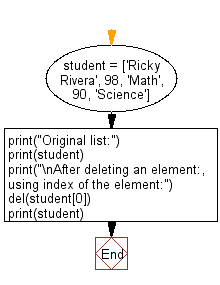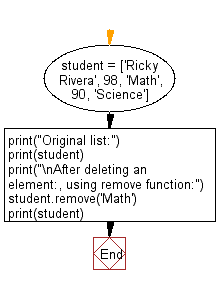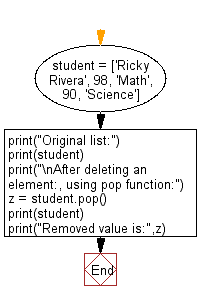﻿ Python: Remove an element from a given list - w3resource# Python: Remove an element from a given list

## Python List: Exercise - 211 with Solution

Write a Python program to remove an element from a given list.

Sample Solution-1:

Python Code:

``````student = ['Ricky Rivera', 98, 'Math', 90, 'Science']
print("Original list:")
print(student)
print("\nAfter deleting an element:, using index of the element:")
del(student)
print(student)
```
```

Sample Output:

```Original list:
['Ricky Rivera', 98, 'Math', 90, 'Science']

After deleting an element:, using index of the element:
[98, 'Math', 90, 'Science']
```

Flowchart:## Visualize Python code execution:

The following tool visualize what the computer is doing step-by-step as it executes the said program:

Sample Solution-2:

Python Code:

``````student = ['Ricky Rivera', 98, 'Math', 90, 'Science']
print("Original list:")
print(student)
print("\nAfter deleting an element:, using remove function:")
student.remove('Math')
print(student)
```
```

Sample Output:

```Original list:
['Ricky Rivera', 98, 'Math', 90, 'Science']

After deleting an element:, using remove function:
['Ricky Rivera', 98, 90, 'Science']
```

Flowchart:## Visualize Python code execution:

The following tool visualize what the computer is doing step-by-step as it executes the said program:

Sample Solution-3:

Python Code:

``````student = ['Ricky Rivera', 98, 'Math', 90, 'Science']
print("Original list:")
print(student)
print("\nAfter deleting an element:, using pop function:")
z = student.pop()
print(student)
print("Removed value is:",z)
```
```

Sample Output:

```Original list:
['Ricky Rivera', 98, 'Math', 90, 'Science']

After deleting an element:, using pop function:
['Ricky Rivera', 98, 'Math', 90]
Removed value is: Science
```

Flowchart:## Visualize Python code execution:

The following tool visualize what the computer is doing step-by-step as it executes the said program:

Python Code Editor:

Have another way to solve this solution? Contribute your code (and comments) through Disqus.

What is the difficulty level of this exercise?

Test your Python skills with w3resource's quiz

﻿

## Python: Tips of the Day

```print(2_000_000)
```2000000